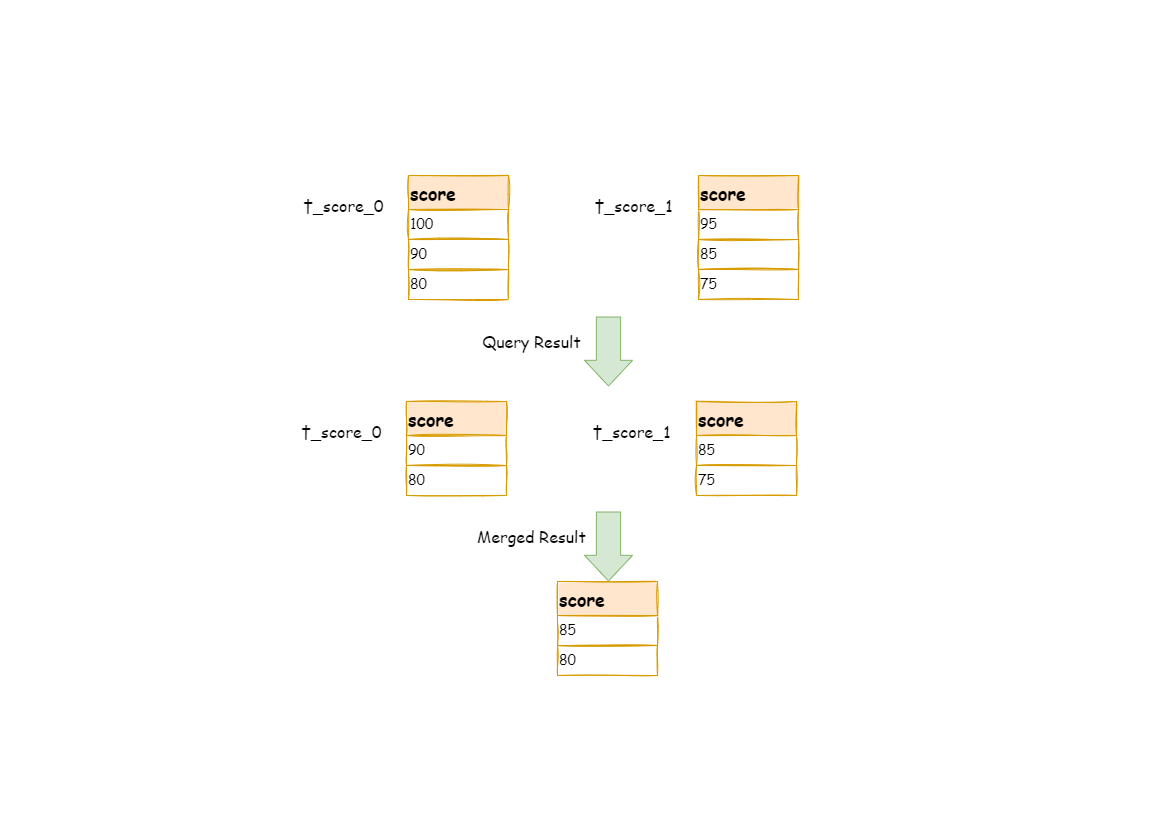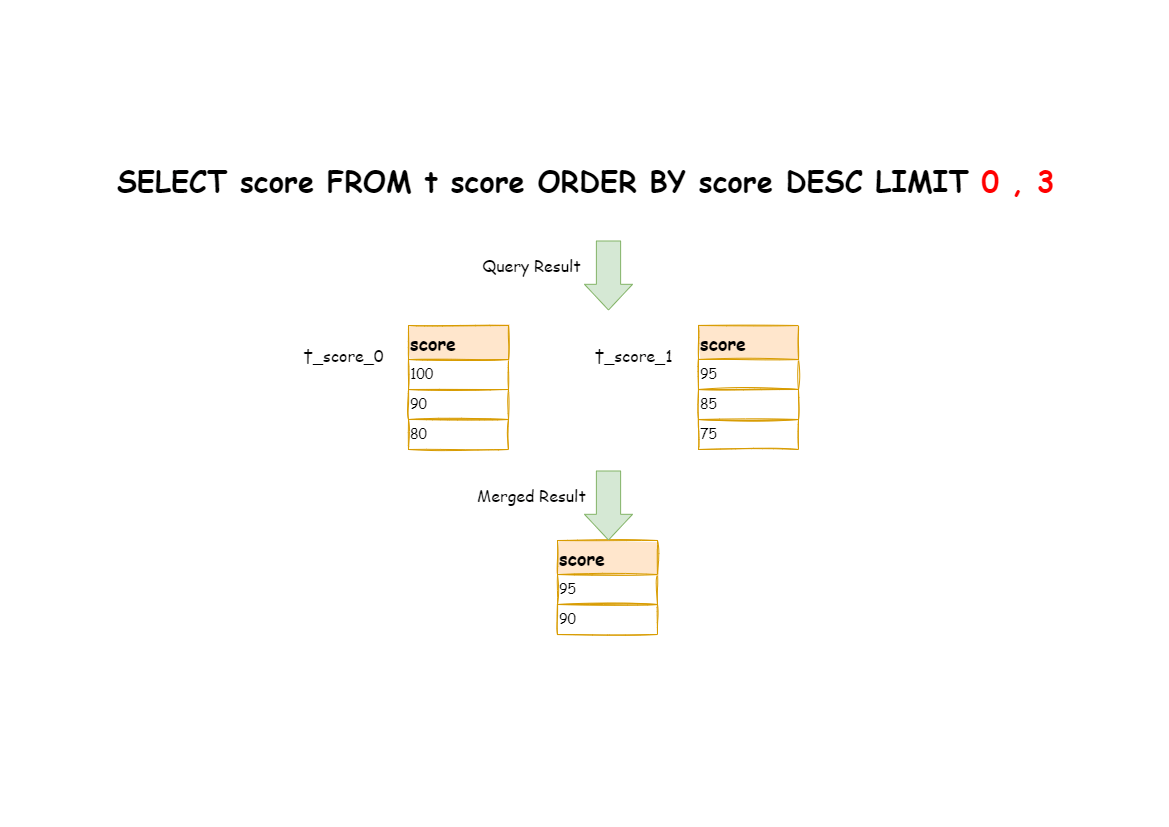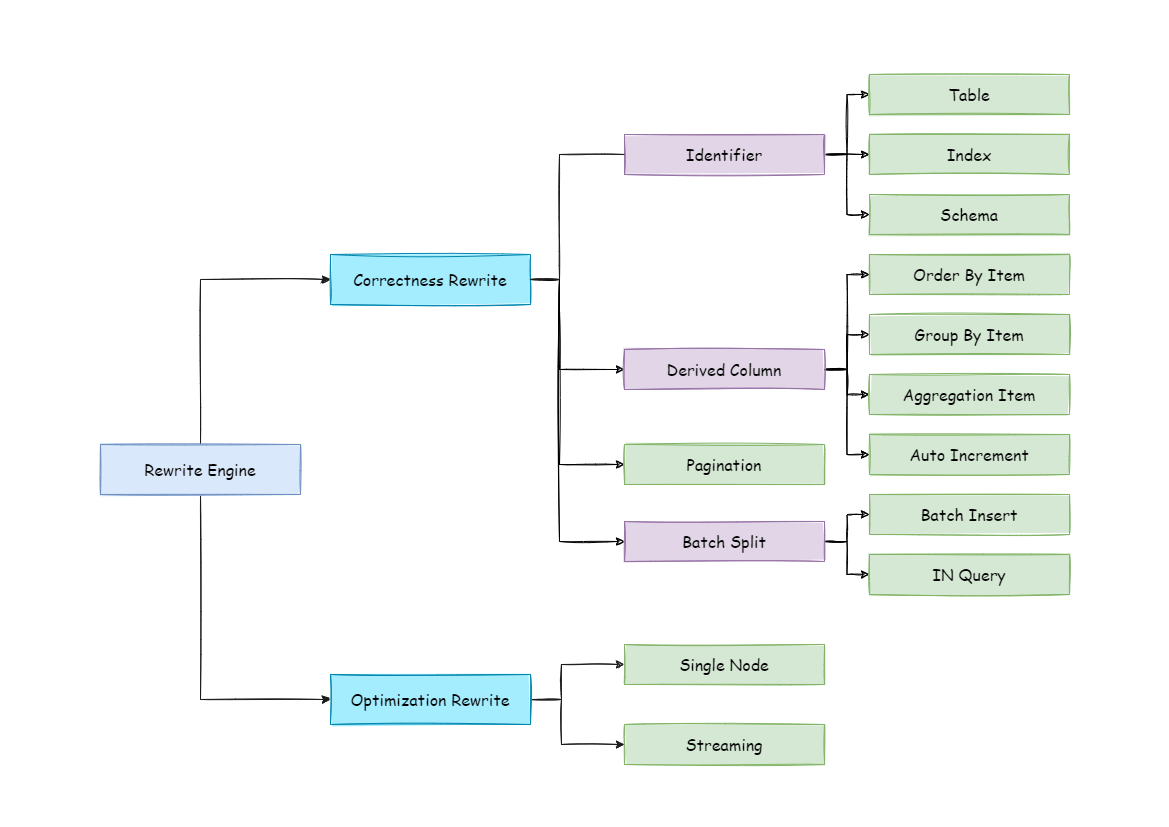Retro Eye care Haitian Deep Dark Default

# Rewrite Engine

SQL written by engineers for logical databases and tables cannot be directly executed in real databases.

SQL rewriting is used to rewrite logical SQL into SQL that can be executed correctly in real databases. It includes rewriting for correctness and rewriting for optimization.

## Rewriting for Correctness

In a scenario with table shards, you need to rewrite the logical table name in the table shards configuration to the real table name obtained after routing.

Only database shards do not require rewriting table names. Additionally, it also includes column derivation and pagination information correction.

## Identifier Rewriting

The identifiers that need to be overwritten include table names, index names, and Schema names.

Rewriting table names is the process of finding the location of the logical table in the original SQL and rewriting it into a real table.

Table name rewriting is a typical scenario that requires SQL parsing. For example, if logical SQL is:

``````SELECT order_id FROM t_order WHERE order_id=1;
``````

Assume that the SQL is configured with the shard key `order_id` and `order_id=1`, it will be routed to shard table 1. Then the rewritten SQL should be:

``````SELECT order_id FROM t_order_1 WHERE order_id=1;
``````

In the simplest SQL scenario, it doesn’t seem to matter whether or not the SQL is parsed into an abstract syntax tree.

SQL can be rewritten correctly only by finding and replacing strings. However, it is impossible to achieve the same effect in the following scenarios.

``````SELECT order_id FROM t_order WHERE order_id=1 AND remarks=' t_order xxx';
``````

The correct rewritten SQL would be:

``````SELECT order_id FROM t_order_1 WHERE order_id=1 AND remarks=' t_order xxx';
``````

``````SELECT order_id FROM t_order_1 WHERE order_id=1 AND remarks=' t_order_1 xxx';
``````

Because there may be characters similar to the table name, you cannot rewrite SQL simply by replacing strings.

Let’s look at a more complex scenario:

``````SELECT t_order.order_id FROM t_order WHERE t_order.order_id=1 AND remarks=' t_order xxx';
``````

The above SQL uses the table name as an identifier of the field, so it needs to be modified when SQL is rewritten:

``````SELECT t_order_1.order_id FROM t_order_1 WHERE t_order_1.order_id=1 AND remarks=' t_order xxx';
``````

If a table alias is defined in SQL, the alias does not need to be modified, even if it is the same as the table name. For example:

``````SELECT t_order.order_id FROM t_order AS t_order WHERE t_order.order_id=1 AND remarks=' t_order xxx';
``````

Rewriting the table name is enough for SQL rewriting.

``````SELECT t_order.order_id FROM t_order_1 AS t_order WHERE t_order.order_id=1 AND remarks=' t_order xxx';
``````

The index name is another identifier that can be rewritten. In some databases (such as MySQL and SQLServer), indexes are created in the dimension of tables.

Indexes in different tables can have the same name. In other databases (such as PostgreSQL and Oracle), indexes are created in the dimension of databases, and even indexes on different tables should have unique names.

In ShardingSphere, schemas are managed in the same way as tables. Logical Schemas are used to manage a set of data sources.

Therefore, ShardingSphere needs to replace the logical Schema written by the user in SQL with the real database Schema.

Currently, ShardingSphere does not support the use of Schema in DQL and DML statements. It only supports the use of Schema in database management statements. For example:

``````SHOW COLUMNS FROM t_order FROM order_ds;
``````

Schema rewriting refers to the rewriting of a logical Schema using unicast routing to a correct and real Schema that is randomly found.

## Column Derivation

There are two cases that need to complement columns in a query statement. In the first case, ShardingSphere needs to get the data during the result merge, but the data is not returned by the queried SQL.

In this case, it mainly applies to GROUP BY and ORDER BY. When merging the results, you need to group and order the field items according to `GROUP BY` and `ORDER BY`, but if the original SQL does not contain grouping or ordering items in the selections, you need to rewrite the original SQL. Let’s look at a scenario where the original SQL has the required information for result merge.

``````SELECT order_id, user_id FROM t_order ORDER BY user_id;
``````

Since `user_id` is used for sorting, the data of `user_id` needs to be retrieved in the result merge. And the above SQL can obtain the data of `user_id`, so there is no need to add columns.

If the selection does not contain the columns required to merge the results, you need to fill the columns, as in the following SQL:

``````SELECT order_id FROM t_order ORDER BY user_id;
``````

Since the original SQL does not contain the `user_id` required in the result merge, you need to fill in and rewrite the SQL. Then SQL would be:

``````SELECT order_id, user_id AS ORDER_BY_DERIVED_0 FROM t_order ORDER BY user_id;
``````

It should be noted that only missing columns are complemented instead of all columns. And SQL that contains * in the SELECT statement will also selectively complement columns based on the metadata information of the table. Here is a relatively complex column derivation scenario of SQL:

``````SELECT o.* FROM t_order o, t_order_item i WHERE o.order_id=i.order_id ORDER BY user_id, order_item_id;
``````

We assume that only the table `t_order_item` contains the column `order_item_id`. According to the metadata information of the table, when the result is merged, the `user_id` in the ordering items exists on the table `t_order`, so there is no need to add columns. `order_item_id` is not in `t_order`, so column derivation is required. Then SQL would become:

``````SELECT o.*, order_item_id AS ORDER_BY_DERIVED_0 FROM t_order o, t_order_item i WHERE o.order_id=i.order_id ORDER BY user_id, order_item_id;
``````

The second case of column derivation is the use of AVG aggregate functions. In distributed scenarios, using (avg1 + avg2 + avg3)/3 to calculate the average is incorrect and should be rewritten as (sum1 + sum2 + sum3) /(count1 + count2 + count3). In this case, rewriting the SQL containing AVG to SUM and COUNT is required, and recalculating the average when the results are merged. For example:

``````SELECT AVG(price) FROM t_order WHERE user_id=1;
``````

The above SQL should be rewritten as:

``````SELECT COUNT(price) AS AVG_DERIVED_COUNT_0, SUM(price) AS AVG_DERIVED_SUM_0 FROM t_order WHERE user_id=1;
``````

Then you can calculate the average correctly by merging the results.

The last type of column derivation is the one that does not need to write the primary key field if the database auto-increment primary key is used during executing an INSERT SQL statement. However, the auto-increment primary key of the database cannot meet the unique primary key in distributed scenarios. Therefore, ShardingSphere provides the generation strategy of the distributed auto-increment primary key. Users can replace the existing auto-increment primary key transparently with the distributed auto-increment primary key without changing the existing code through column derivation. The generation strategy for distributed auto-increment primary keys is described below, and here only SQL rewriting is illustrated. For example, if the primary key of table `t_order` is `order_id`, the original SQL would be:

``````INSERT INTO t_order (`field1`, `field2`) VALUES (10, 1);
``````

As you can see, the above SQL does not contain the auto-increment primary key, which requires the database itself to fill. After ShardingSphere is configured with the auto-increment primary key, SQL will be rewritten as:

``````INSERT INTO t_order (`field1`, `field2`, order_id) VALUES (10, 1, xxxxx);
``````

The rewritten SQL will add column names of the primary key and auto-increment primary key values generated automatically at the end of the INSERT FIELD and INSERT VALUE. The xxxxx in the above SQL represents the auto-increment primary key value generated automatically.

If the INSERT SQL does not contain the column name of the table, ShardingSphere can also compare the number of parameters and the number of columns in the table meta information and automatically generate auto-increment primary keys. For example, the original SQL is:

``````INSERT INTO t_order VALUES (10, 1);
``````

The rewritten SQL will simply add the auto-increment primary key in the column order in which the primary key locates:

``````INSERT INTO t_order VALUES (xxxxx, 10, 1);
``````

If you use placeholders to write SQL, you only need to rewrite the parameter list, not the SQL itself.

## Pagination Correction

The scenario of acquiring pagination data from multiple databases is different from that of one single database. If every 10 pieces of data are taken as one page, the user wants to take the second page of data. It is not correct to acquire `LIMIT 10, 10` under sharding situations, or take out the first 10 pieces of data according to sorting conditions after merging. For example, if SQL is:

``````SELECT score FROM t_score ORDER BY score DESC LIMIT 1, 2;
``````

The following picture shows the pagination execution results without SQL rewriting.As shown in the picture, if you want to acquire the second and the third piece of data sorted by score in both tables, and they are supposed to be `95` and `90`.

Since executed SQL can only acquire the second and the third piece of data from each table, i.e., `90` and `80` from `t_score_0`, `85` and `75` from `t_score_1`. When merging results, it can only merge from `90`, `80`, `85` and `75` already acquired, so the right result cannot be acquired anyway.

The right way is to rewrite pagination conditions as `LIMIT 0, 3`, take out all the data from the first two pages and calculate the right data based on sorting conditions. The following picture shows the execution results of pagination after SQL rewrite.The latter the offset position is, the lower the efficiency of using `LIMIT` pagination will be. There are many ways to avoid using `LIMIT` as pagination method, such as constructing a secondary index to the number of line records and line offsets or using the end ID of the last pagination data as a condition for the next query.

When revising pagination information, if the users use the placeholder to write SQL, they only need to rewrite the parameter list rather than SQL itself.

## Batch Split

When using bulk inserted SQL, if the inserted data crosses shards, the SQL needs to be rewritten to prevent excess data from being written to the database.

The insertion operation differs from the query operation in that the query statement does not affect the data even if it uses the shard key that does not exist in the current shard. In contrast, insertion operations must remove excess shard keys. For example, see the following SQL:

``````INSERT INTO t_order (order_id, xxx) VALUES (1, 'xxx'), (2, 'xxx'), (3, 'xxx');
``````

If the database is still divided into two parts according to the odd and even number of order_id, this SQL will be executed after its table name is revised. Then, both shards will be written with the same record.

Though only the data that satisfies sharding conditions can be retrieved from the query statement, it is not reasonable for the schema to have excessive data. So SQL should be rewritten as:

``````INSERT INTO t_order_0 (order_id, xxx) VALUES (2, 'xxx');
INSERT INTO t_order_1 (order_id, xxx) VALUES (1, 'xxx'), (3, 'xxx');
``````

IN query is similar to batch insertion, but IN operation will not lead to wrong data query result. Through rewriting IN query, the query performance can be further improved. See the following SQL:

``````SELECT * FROM t_order WHERE order_id IN (1, 2, 3);
``````

The SQL is rewritten as：

``````SELECT * FROM t_order_0 WHERE order_id IN (2);
SELECT * FROM t_order_1 WHERE order_id IN (1, 3);
``````

The query performance will be further improved. For now, ShardingSphere has not realized this rewrite strategy, so the current rewrite result is:

``````SELECT * FROM t_order_0 WHERE order_id IN (1, 2, 3);
SELECT * FROM t_order_1 WHERE order_id IN (1, 2, 3);
``````

Though the execution result of SQL is right, it did not achieve the highest query efficiency.

## Rewriting for Optimization

Its purpose is to effectively improve performance without influencing the correctness of the query. It can be divided into single node optimization and stream merger optimization.

### Single Node Optimization

It refers to the optimization that stops the SQL rewrite from the route to the single node. After acquiring one route result, if it is routed to a single data node, there is no need to involve result merger, as well as rewrites such as column derivation and pagination information correction.

In particular, there is no need to read from the first piece of information, which reduces the pressure on the database to a large extent and saves meaningless consumption of the network bandwidth.

### Stream Merger Optimization

It only adds `ORDER BY` and ordering items and sorting orders identical with grouping items to SQL that contains `GROUP BY`. And it is used to transfer memory merger to stream merger. Stream merger and memory merger will be explained in detail in the result merger section.

The overall structure of the rewrite engine is shown in the following picture.